Published: 18 September 2015

# Research on friction parameter identification under the influence of vibration and collision

Qiang Chen1
Yingjun Wang2
Ying Chen3
1, 2Department of Control and System Engineering, Nanjing University, 210093, Nanjing, P. R. China
3Department of Management Science and Engineering, Nanjing University, 210093, Nanjing, P. R. China
Corresponding Author:
Yingjun Wang
Views 25

#### Abstract

Vibration and collision in the process of friction will cause large deviation of identification result with the actual situation. In this paper, friction process with the influence of vibration and collision as well as data collection of this process are implemented; to eliminate influence of vibration and collision in the aspect of the original data, sine filter is introduced into friction model according to the theory of Fourier series; ideas of simulated annealing is introduced into genetic algorithm to form hybrid algorithm, friction parameter can be identified combined with model and test data. The numerical results demonstrate the proposed method has effective identification results for friction process under the influence of the vibration and collision.

## 1. Introduction

The existence of friction seriously restricts the performance of the mechanical system, so using appropriate method to identify friction parameter and to make friction compensation has vital significance. Friction process has obvious non-linear features, and it always includes the influence of additional factors such as vibration and collision. Vibration of the projectile and collision between projectile and channel in the process of pushing projectile is an example. This situation increases the recognition difficulty and reduces the precision of friction parameter identification to some extent. Thus, it has significant meaning for the improvement of precision of model parameter identification to use appropriate method to eliminate the additional effect of vibration and collision on friction process.

Due to influence of additional factors, test data under vibration and collision cannot be directly applied to model parameter identification. Meanwhile, the traditional filtering methods always lead to the missing of useful information, which result in identification result cannot be well reflect the performance of the actual friction process. And it is very adverse for the subsequent friction compensation. Precision of model parameter identification determines the credibility in solving practical problems by using the model to a large degree, so choice of identification algorithm is also very important in addition to the model. Genetic algorithm and simulated annealing algorithm are two excellent intelligent algorithm in recent years. They both have a superior capacity to generate and search solution set, but they also have their own insurmountable defects which limit the application of algorithm. Fortunately, the complementarity in performance makes it possible to combine the two algorithms. Frictional experiment with vibration and collision is designed in this paper, and test data can be collected by high-speed photography technology and acceleration sensor. According to the theory of Fourier series, sine filter is introduced into the classical stribeck friction model to eliminate the influence of vibration and collision. At last, with friction model and test data, friction parameter can be identified by hybrid algorithm. The numerical results demonstrate the effectiveness of the identification method.

## 2. Identification model

### 2.1. Friction device

Vibration and collision in process of friction will result in a large error in identification result. In order to further improve the precision of friction parameter identification under the impact of vibration and collision, friction process under the influence of vibration and collision is implemented in this paper based on friction mechanism. The experimental device is shown in Fig. 1. The numbers 1 to 3 respectively represent slippery course, slider and spring impact hammer. The spring impact hammer can release impact energy of 2 J, this energy can make the slider get an initial velocity, and then the slider will move alone the slippery course at a certain speed. In the process of moving, the slider gets not only friction force from slippery course, but also includes its own vibration and collision with the slippery course. In the moving process of the slider, a high speed camera and an acceleration sensor are used to record dynamic data information, after filtering and noise reduction, test data can be used to identify friction parameters in combination with friction model and intelligent algorithm.

Fig. 1Schematic diagram of friction device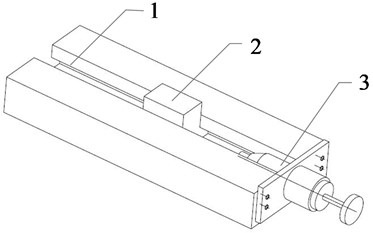### 2.2. Identification model

Friction model can be divided into static friction model and dynamic friction model. Static friction model recognize friction as function of relative velocity between two contact surfaces, which cam well reflect friction process with viscous effect, and it is also the basis of research on parameter identification of dynamic friction model. Stribeck friction model is one of the classic models, this model can reflect most of the friction phenomena, can be used to analyze complex friction phenomena, so it is used widely, which is shown as Eq. (1):

1
$f\left(\nu \right)={f}_{c}+\left({f}_{s}-{f}_{c}\right){e}^{\left[-\left(\frac{\nu }{{\nu }_{s}}\right)\right]}+{f}_{v}v,$

where ${f}_{c}$ is coulomb friction, ${f}_{s}$ is static friction, ${f}_{v}$ is viscous friction and ${V}_{s}$ is stribeck velocity.

Related studies have shown that when vibration and the collision are included into friction process, such as vibration of the projectile and collision between projectile and channel in the process of pushing projectile, classic friction model cannot be well reflect the dynamic performance and static performance of the actual friction process, and it is also very adverse for the subsequent friction compensation. According to the theory of Fourier series, sine filter is introduced into the classical stribeck friction model, in order to eliminate the additional influence of vibration and collision in the aspect of the original data. And then the model can be shown as Eq. (2):

2
$f\left(\nu \right)={f}_{c}+\left({f}_{s}-{f}_{c}\right){e}^{\left[-\left(\frac{\nu }{{\nu }_{s}}\right)\right]}+{f}_{v}v+\sum _{i=1}^{n}{f}_{i}\mathrm{s}\mathrm{i}\mathrm{n}\left({\omega }_{i}\mathrm{t}+{\phi }_{i}\right).$

A precise model is got when $n\to \infty$, considering the feasibility of calculation, set $n$ as 2. There are ten parameters need to be identified in Eq. (2).

## 3. Identification algorithms

### 3.1. Genetic algorithm (GA) and simulated annealing algorithm (SA)

The theory basis for genetic algorithm is schemata theorem and building block hypothesis. Schemata theorem makes genetic algorithm has the possibility to find the global optimal solution, while building block hypothesis points out that the specific pattern can generate the global optimal solution under the effect of genetic operators. High robustness of genetic algorithm make it becomes a new way to solve nonlinear optimization problem.

Simulated annealing algorithm simulates the process of solid annealing. Because of design and application of Metropolis rule, SA can accept worse solution in the process of iterations at a certain probability to avoid SA is trapped into local optimal solution. And this makes sure that SA can obtain the optimal solution at a great possibility when to solve combinatorial optimization, system identification and other issues.

### 3.2. Simulated annealing genetic algorithm (SGA)

Genetic algorithm and simulated annealing algorithm has attracted much attention in recent years, and has been widely used in various fields. However, single intelligent algorithm always has its own limitations, such as the convergence speed of genetic algorithm is slow and its local search ability is not very good; at the same time, optimization time of simulated annealing algorithm is always very long. Anyway, these two intelligent algorithms are important but not powerful search methods.

Genetic algorithm and simulated annealing algorithm are complementary in optimize performance, and simulated annealing genetic algorithm is a hybrid algorithm which combined with that two intelligent algorithms. The performance of that hybrid algorithm gets a big promotion because of it possesses advantages of both that two algorithms, and just because of this, that hybrid algorithm is widely used in various fields such as system identification. Hybrid algorithm introduces ideas of simulated annealing while sets genetic algorithm as basic framework, which flowchart is shown in Fig. 2.

Fig. 2Simulated annealing genetic algorithm flowchart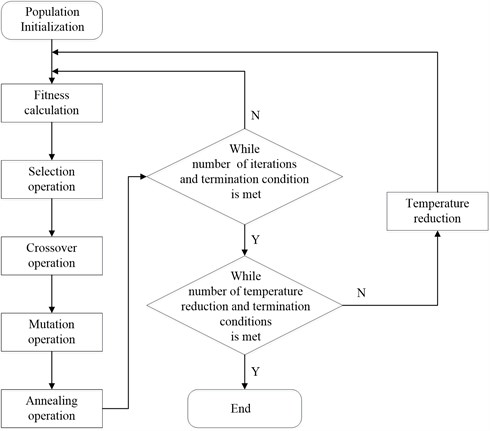## 4. Case study

### 4.1. Experimental installation

In this study, a spring shock device is adopted to simulate the friction process under influence of vibration and collision, which is shown in Fig. 1. The spring impact hammer with impact energy of 2 J is put in initial position. Then the slider is driven by the spring impact hammer and moves alone the slippery course at a certain speed. The variation rule of acceleration of the slider during the friction process was recorded by acceleration sensor and data acquisition system, while the displacement data and velocity information is measured by a high speed camera. In addition, a LED cold light source is used to solve the problem of illumination compensation. The actual installation of measuring equipment is shown in Fig. 3.

Fig. 3Schematic diagram of equipment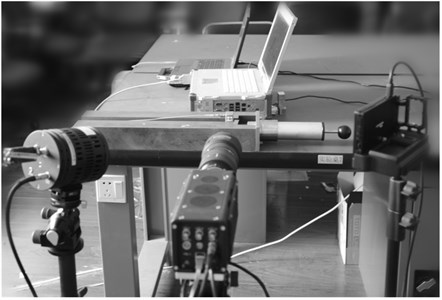### 4.2. Data processing

During the friction process, displacement information of the slider is recorded by a high speed camera while the sampling frequency is set at about 4 K Hz. The information what we are concerned is velocity single and acceleration single, however, the high speed camera can only get displacement information, so two differential operation of displacement need to be executed to obtain single of velocity and acceleration. Then, the friction force time curve can be obtained combining with the quality of the solider. During the experiment, there would be noise interference which can easily cause data distortion, thus low-pass filter is taken to filter the original data before differential operation so that high frequency noise can be restrained effectively and accuracy of data can be ensured.

The velocity curve and acceleration-time diagram during the solider moving alone the slippery course are respectively shown in Fig. 4 and Fig. 5.

Fig. 4Velocity curve of solider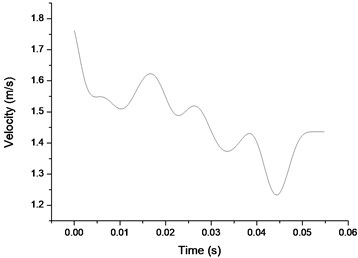Fig. 5Acceleration-time diagram of solider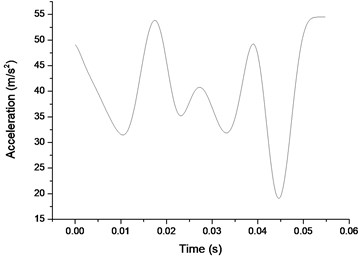### 4.3. Parameter identification without sine filter

Parameter identification can be performed with simulated annealing genetic algorithm, and the identification results are shown in Fig. 6 and Table 1.

Fig. 6Actual friction and theory friction comparison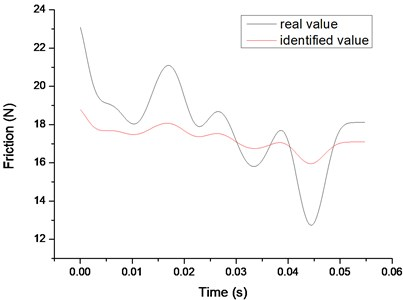Table 1Identification result without sine filter

 Friction (N) ${F}_{c}$ ${F}_{s}$ ${F}_{v}$ ${V}_{s}$ Identified value 10.0000 17.8104 5.0000 0.2512

### 4.4. Parameter identification with sine filter

Parameter identification can be performed with simulated annealing genetic algorithm, and the identification results are shown in Fig. 7 and Table 2.

Fig. 7Actual friction and theory friction comparison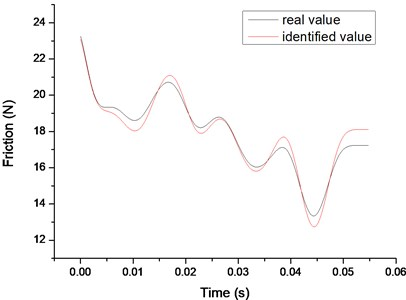Table 2Identification result without sine filter

 Items ${F}_{c}$ (N) ${F}_{s}$ (N) ${F}_{v}$ (N∙m/s) ${V}_{s}$ (m/s) ${F}_{a}$ (N) Value 4.0266 12.7125 14.7817 1.9551 1.5971 Items ${F}_{b}$ (N) ${\mathrm{\Omega }}_{a}$ (rad/s) ${\mathrm{\Omega }}_{b}$ (rad/s) ${\mathrm{\Phi }}_{a}$ (rad/s) ${\mathrm{\Phi }}_{b}$ (rad/s) Value 0.2665 2.8556 0.7038 0.3363 0.8242

## 5. Conclusions

Sine filter is included into classic friction model in this paper, which can express additional interference signal to some extent. And it can be used to eliminate the influence of interference signal in the aspect of the original data, which can avoid information distortion caused by the traditional filtering method. The introduction of sine filter can improve precision of parameter identification, and the effectiveness of this method is confirmed by identifying the model parameters with SGA. However, the hybrid algorithm also has its disadvantage such as long execution time, thus further work of this study would do more research to improve algorithm efficiency.

Accepted
24 July 2015
Published
18 September 2015
SUBJECTS
Fault diagnosis based on vibration signal analysis
Keywords
friction parameters identification
vibration and collision
sine filter
hybrid algorithm
Acknowledgements

This is supported by the National Key Scientific Instrument Development Projects, No. 2013YQ470765.